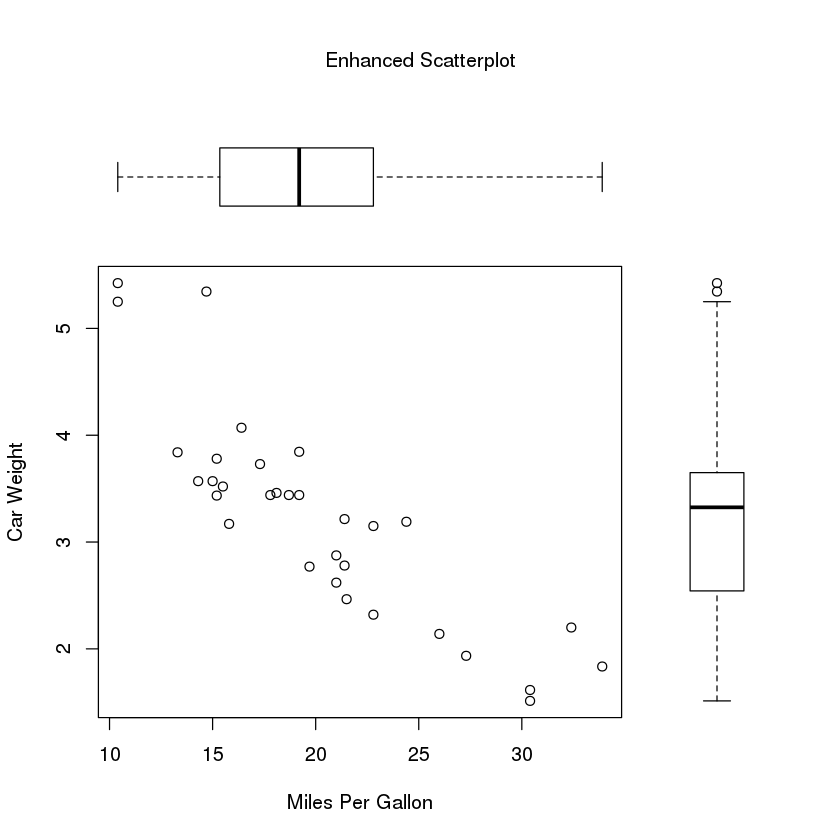In :
#@author: Venky Rao raove@us.ibm.com
#@last edited: 20 Aug 2017
#@source: materials, data and examples adapted from R in Action 2nd Edition by Dr. Robert Kabacoff


Working with graphs¶

In :
#In R, in a typical interactive session, you build a graph, one statement at a time, adding features, until you have what you want
#here's an example:

In :
attach(mtcars) #attach the mtcars dataset that comes with R
mtcars #view the mtcars dataset

mpgcyldisphpdratwtqsecvsamgearcarb
Mazda RX421.0 6 160.0110 3.90 2.62016.460 1 4 4
Mazda RX4 Wag21.0 6 160.0110 3.90 2.87517.020 1 4 4
Datsun 71022.8 4 108.0 93 3.85 2.32018.611 1 4 1
Hornet 4 Drive21.4 6 258.0110 3.08 3.21519.441 0 3 1
Hornet Sportabout18.7 8 360.0175 3.15 3.44017.020 0 3 2
Valiant18.1 6 225.0105 2.76 3.46020.221 0 3 1
Duster 36014.3 8 360.0245 3.21 3.57015.840 0 3 4
Merc 240D24.4 4 146.7 62 3.69 3.19020.001 0 4 2
Merc 23022.8 4 140.8 95 3.92 3.15022.901 0 4 2
Merc 28019.2 6 167.6123 3.92 3.44018.301 0 4 4
Merc 280C17.8 6 167.6123 3.92 3.44018.901 0 4 4
Merc 450SE16.4 8 275.8180 3.07 4.07017.400 0 3 3
Merc 450SL17.3 8 275.8180 3.07 3.73017.600 0 3 3
Merc 450SLC15.2 8 275.8180 3.07 3.78018.000 0 3 3
Cadillac Fleetwood10.4 8 472.0205 2.93 5.25017.980 0 3 4
Lincoln Continental10.4 8 460.0215 3.00 5.42417.820 0 3 4
Chrysler Imperial14.7 8 440.0230 3.23 5.34517.420 0 3 4
Fiat 12832.4 4 78.7 66 4.08 2.20019.471 1 4 1
Honda Civic30.4 4 75.7 52 4.93 1.61518.521 1 4 2
Toyota Corolla33.9 4 71.1 65 4.22 1.83519.901 1 4 1
Toyota Corona21.5 4 120.1 97 3.70 2.46520.011 0 3 1
Dodge Challenger15.5 8 318.0150 2.76 3.52016.870 0 3 2
AMC Javelin15.2 8 304.0150 3.15 3.43517.300 0 3 2
Camaro Z2813.3 8 350.0245 3.73 3.84015.410 0 3 4
Pontiac Firebird19.2 8 400.0175 3.08 3.84517.050 0 3 2
Fiat X1-927.3 4 79.0 66 4.08 1.93518.901 1 4 1
Porsche 914-226.0 4 120.3 91 4.43 2.14016.700 1 5 2
Lotus Europa30.4 4 95.1113 3.77 1.51316.901 1 5 2
Ford Pantera L15.8 8 351.0264 4.22 3.17014.500 1 5 4
Ferrari Dino19.7 6 145.0175 3.62 2.77015.500 1 5 6
Maserati Bora15.0 8 301.0335 3.54 3.57014.600 1 5 8
Volvo 142E21.4 4 121.0109 4.11 2.78018.601 1 4 2
In :
plot(wt, mpg) #generates a scatter plot between automobile weight (x-axis) and miles per gallon (y-axis)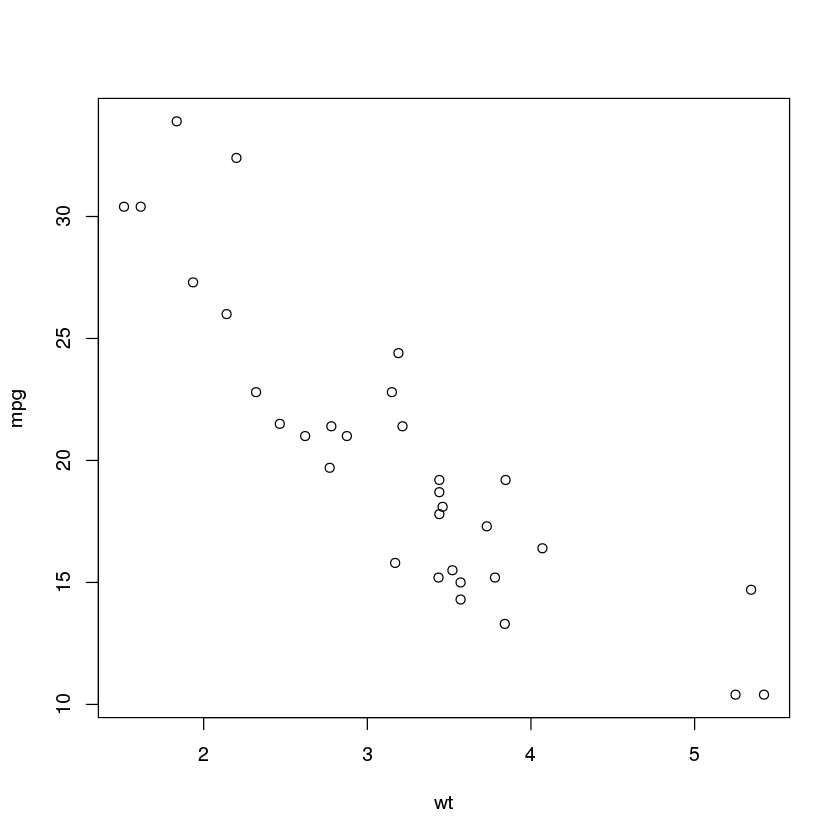In :
plot(wt, mpg) #generates a scatter plot between automobile weight (x-axis) and miles per gallon (y-axis)
abline(lm(mpg~wt)) #adds a line of best fit to the chart created above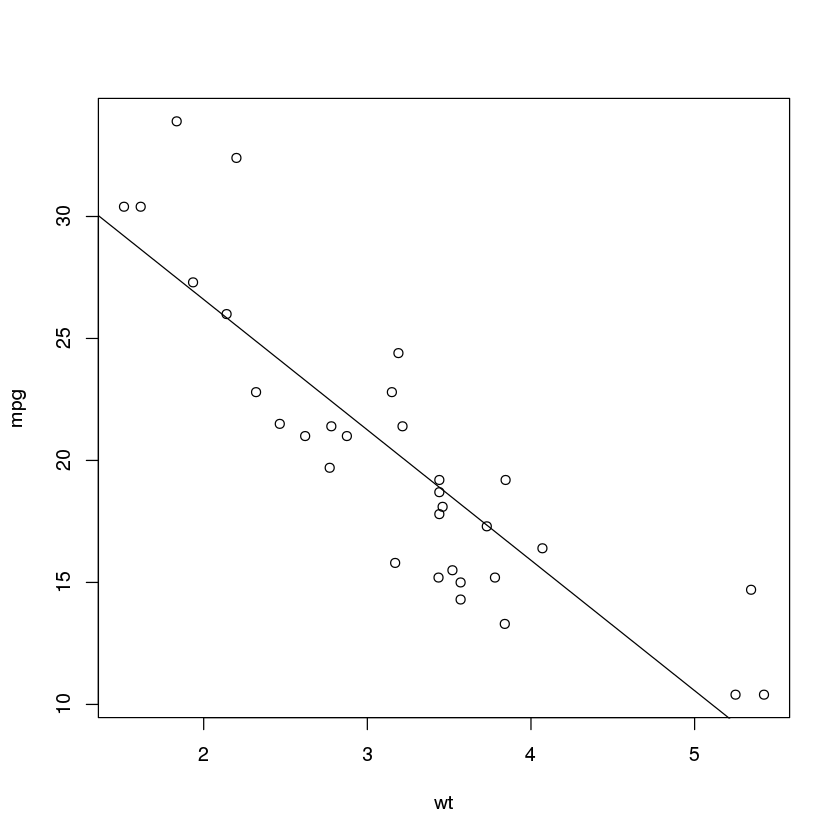In :
plot(wt, mpg) #generates a scatter plot between automobile weight (x-axis) and miles per gallon (y-axis)
abline(lm(mpg~wt)) #adds a line of best fit to the chart created above
title("Regression of MPG on Weight") #adds a title to the chart
detach(mtcars) #detaches the mtcars data frame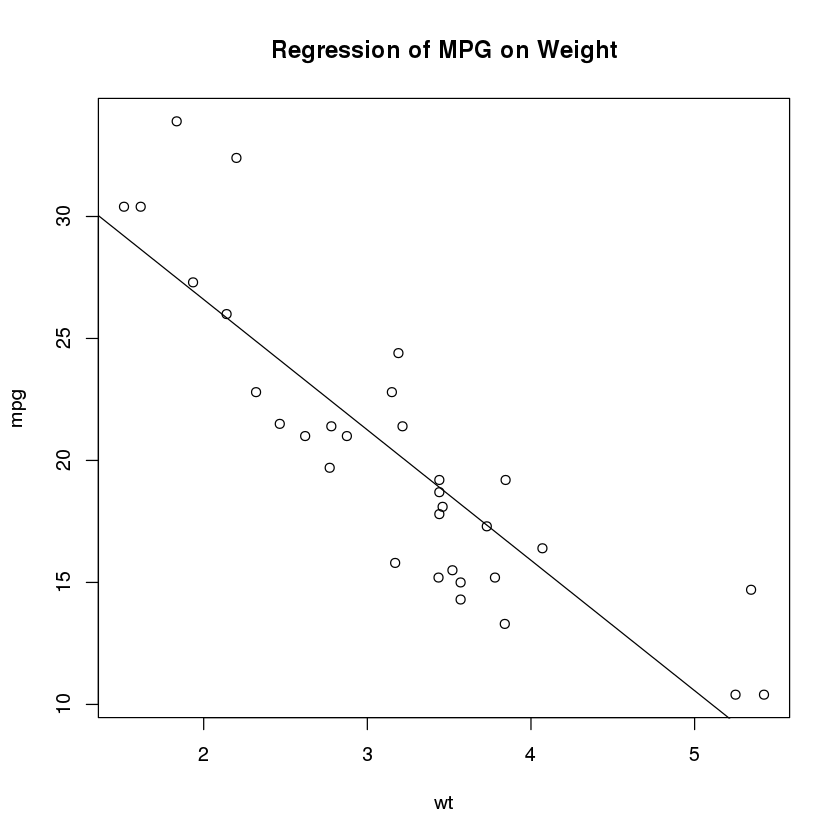A simple example¶

In :
#let's create some data for our graph
dose <- c(20, 30, 40, 45, 60)
drugA <- c(16, 20, 27, 40, 60)
drugB <- c(15, 18, 25, 31, 40)

In :
#a simple line graph relating dose to drugA can be generated as follows:
plot(dose, drugA, type = "b") # type = "b" means both points and a line should be plotted
#explore help(plot) for more information on all parameters that can be specified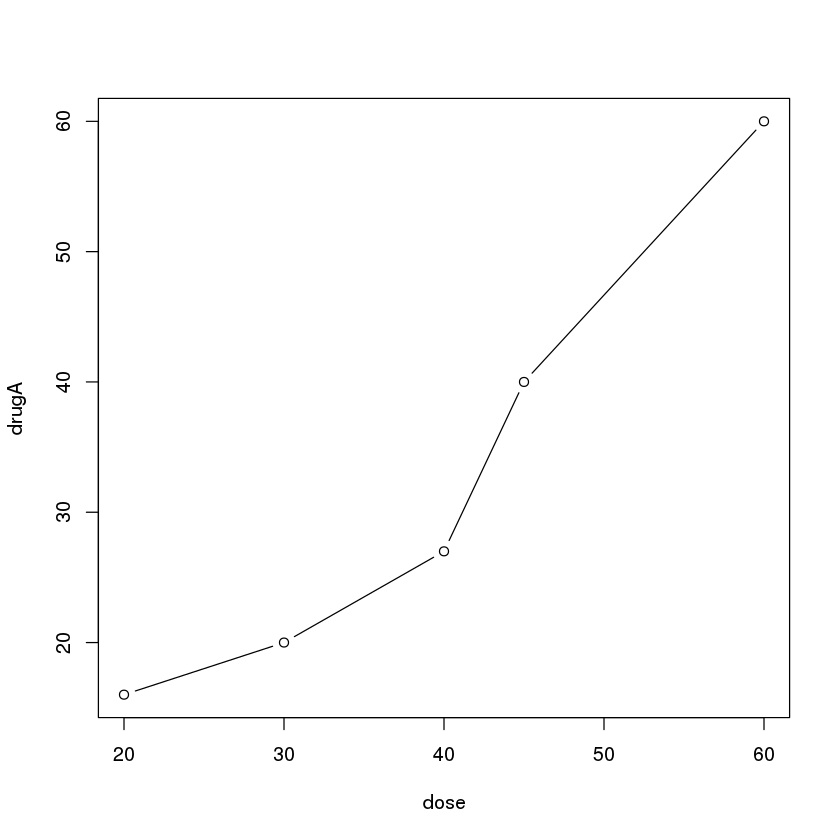Graphical parameters¶

In :
#you can customize many features of a graph (eg fonts, colors, axes, labels, etc)
#one way of doing this is by using the par() function
#values set using the par() function will be in effect for the rest of session or until they're changed

In :
#specifying par() without any parameters produces a list of the current graphical settings
par()
#length(par()) == 72

$xlog FALSE$ylog
FALSE
$adj 0.5$ann
TRUE
$ask FALSE$bg
'white'
$bty 'o'$cex
1
$cex.axis 1$cex.lab
1
$cex.main 1.2$cex.sub
1
$cin 1. 0.15 2. 0.2$col
'black'
$col.axis 'black'$col.lab
'black'
$col.main 'black'$col.sub
'black'
$cra 1. 10.8 2. 14.4$crt
0
$csi 0.2$cxy
1. 0.0276412776412776
2. 0.0414364640883978
$din 1. 6.66666666666667 2. 6.66666666666667$err
0
$family ''$fg
'black'
$fig 1. 0 2. 1 3. 0 4. 1$fin
1. 6.66666666666667
2. 6.66666666666667
$font 1$font.axis
1
$font.lab 1$font.main
2
$font.sub 1$lab
1. 5
2. 5
3. 7
$las 0$lend
'round'
$lheight 1$ljoin
'round'
$lmitre 10$lty
'solid'
$lwd 1$mai
1. 1.02
2. 0.82
3. 0.82
4. 0.42
$mar 1. 5.1 2. 4.1 3. 4.1 4. 2.1$mex
1
$mfcol 1. 1 2. 1$mfg
1. 1
2. 1
3. 1
4. 1
$mfrow 1. 1 2. 1$mgp
1. 3
2. 1
3. 0
$mkh 0.001$new
FALSE
$oma 1. 0 2. 0 3. 0 4. 0$omd
1. 0
2. 1
3. 0
4. 1
$omi 1. 0 2. 0 3. 0 4. 0$page
TRUE
$pch 1$pin
1. 5.42666666666667
2. 4.82666666666667
$plt 1. 0.123 2. 0.937 3. 0.153 4. 0.877$ps
12
$pty 'm'$smo
1
$srt 0$tck
NA
$tcl -0.5$usr
1. 0
2. 1
3. 0
4. 1
$xaxp 1. 0 2. 1 3. 5$xaxs
'r'
$xaxt 's'$xpd
FALSE
$yaxp 1. 0 2. 1 3. 5$yaxs
'r'
$yaxt 's'$ylbias
0.2
In :
#adding no.readonly = T to the par() function produces a list of settings that can be modified

$xlog FALSE$ylog
FALSE
$adj 0.5$ann
TRUE
$ask FALSE$bg
'white'
$bty 'o'$cex
1
$cex.axis 1$cex.lab
1
$cex.main 1.2$cex.sub
1
$col 'black'$col.axis
'black'
$col.lab 'black'$col.main
'black'
$col.sub 'black'$crt
0
$err 0$family
''
$fg 'black'$fig
1. 0
2. 1
3. 0
4. 1
$fin 1. 6.66666666666667 2. 6.66666666666667$font
1
$font.axis 1$font.lab
1
$font.main 2$font.sub
1
$lab 1. 5 2. 5 3. 7$las
0
$lend 'round'$lheight
1
$ljoin 'round'$lmitre
10
$lty 'solid'$lwd
1
$mai 1. 1.02 2. 0.82 3. 0.82 4. 0.42$mar
1. 5.1
2. 4.1
3. 4.1
4. 2.1
$mex 1$mfcol
1. 1
2. 1
$mfg 1. 1 2. 1 3. 1 4. 1$mfrow
1. 1
2. 1
$mgp 1. 3 2. 1 3. 0$mkh
0.001
$new FALSE$oma
1. 0
2. 0
3. 0
4. 0
$omd 1. 0 2. 1 3. 0 4. 1$omi
1. 0
2. 0
3. 0
4. 0
$pch 1$pin
1. 5.42666666666667
2. 4.82666666666667
$plt 1. 0.123 2. 0.937 3. 0.153 4. 0.877$ps
12
$pty 'm'$smo
1
$srt 0$tck
NA
$tcl -0.5$usr
1. 0
2. 1
3. 0
4. 1
$xaxp 1. 0 2. 1 3. 5$xaxs
'r'
$xaxt 's'$xpd
FALSE
$yaxp 1. 0 2. 1 3. 5$yaxs
'r'
$yaxt 's'$ylbias
0.2
In :
opar <- par(no.readonly = T) #save the list of settings that can be modified to the "opar" object
par(lty = 2, pch = 17) # lty = 2 means line type dashed; pch = 17 means solid triangle as plotting symbol
plot(dose, drugA, type = "b") #plotting the chart again with new parameters
par(opar) #restore original settings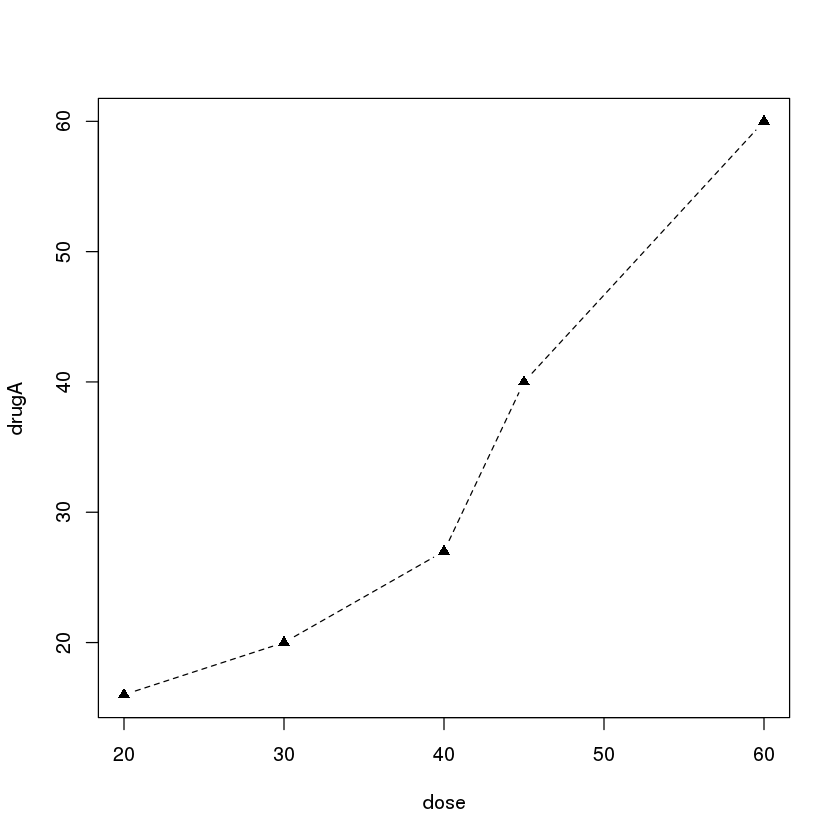In :
#another way of generating the same graph is to specify the options directly into the main plotting function
#in this case, the options are only in effect for this specific graph
plot(dose, drugA, type = "b", lty = 2, pch = 17)Symbols and lines¶

In :
plot(dose, drugA, type = "b", lty = 3, lwd = 3, pch = 15, cex = 2) #lwd = line width, cex = symbol size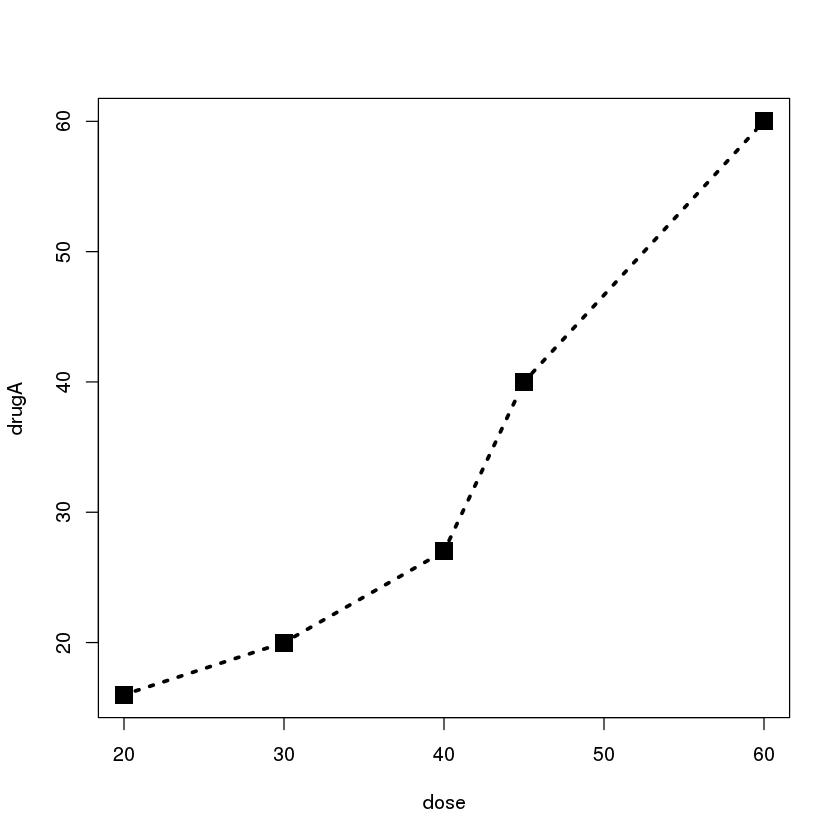Colors¶

In :
#here's some sample code to explore colors in R
n <- 10
mycolors <- rainbow(n) #rainbow() is a function in R that lets you create vectors of contiguous colors
pie(rep(1, n), labels = mycolors, col = mycolors) # rep(1, n) creates a vector of the value "1" repeated "n" times
# pie() creates a pie chart with "n" equal parts with each part having a diff color of the rainbow
mygrays <- gray(0:n/n) # this produces a vector of 10 gray levels
pie(rep(1, n), labels = mygrays, col = mygrays)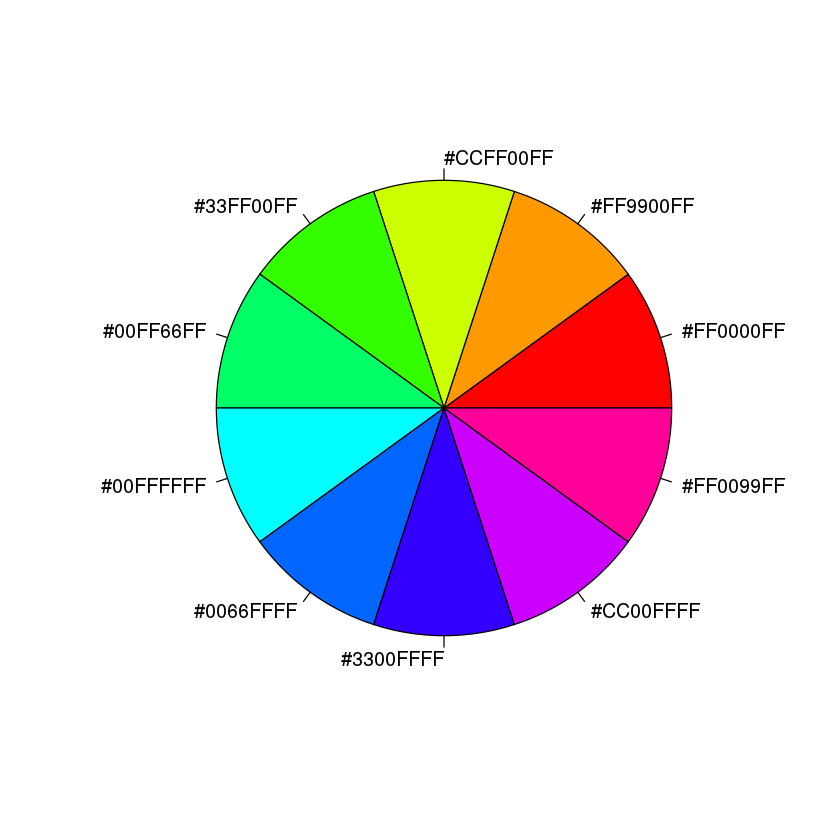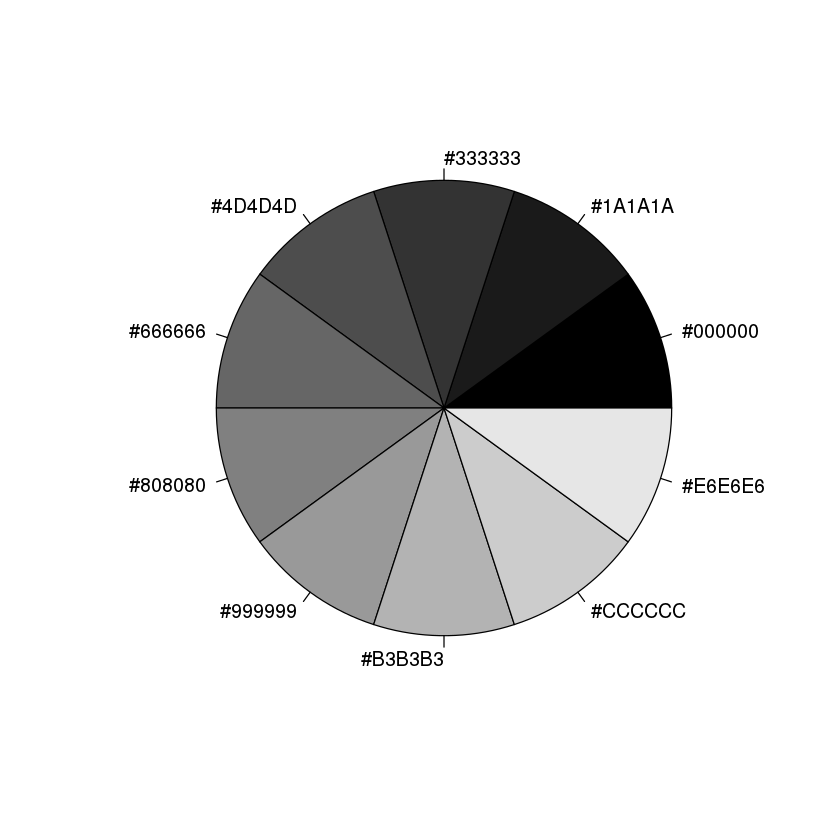Text characteristics, graph and margin dimensions¶

In :
#example of a graph where text characteristics, graph and margin dimenions are customized
#data
dose <- c(20, 30, 40, 45, 60) #vector with dosage levels
drugA <- c(16, 20, 27, 40, 60) #vector with drugA dosage
drugB <- c(15, 18, 25, 31, 40) #vector with drugB dosage

#identify settings that can be customized
opar <- par(no.readonly = T) #save the list of settings that can be modified to the "opar" object

#customize settings
par(pin = c(2, 3)) #plot dimensions (width, height) in inches
par(lwd = 2, cex = 1.5) #lwd = line width, 2x normal size; cex = plotted text, 1.5x normal size
par(cex.axis = 0.75, font.axis = 3) #cex.axis = magnification of axis text relative to cex; font.axis is set to italics

#create the plots
plot(dose, drugA, type = "b", pch = 19, lty = 2, col = "red") #type = line graph; pch = symbol; lty = line type; col = color
plot(dose, drugB, type = "b", pch = 23, lty = 6, col = "blue", bg = "green") #type = line graph; pch = symbol; lty = line type;
#col = color; bg = background color

#restore orginal settings
par(opar) #restore original settings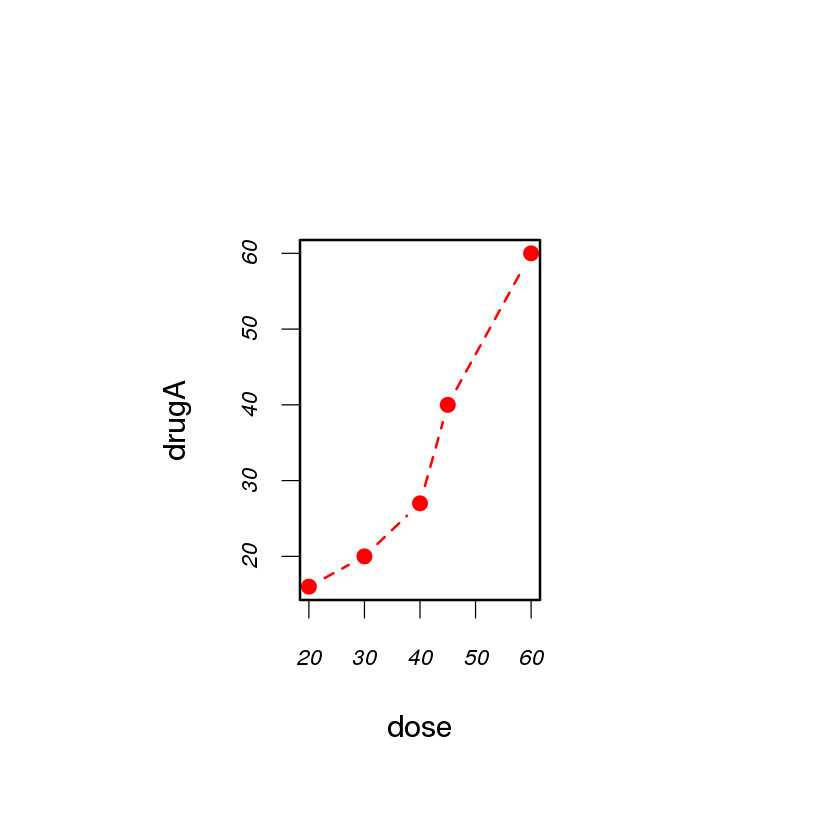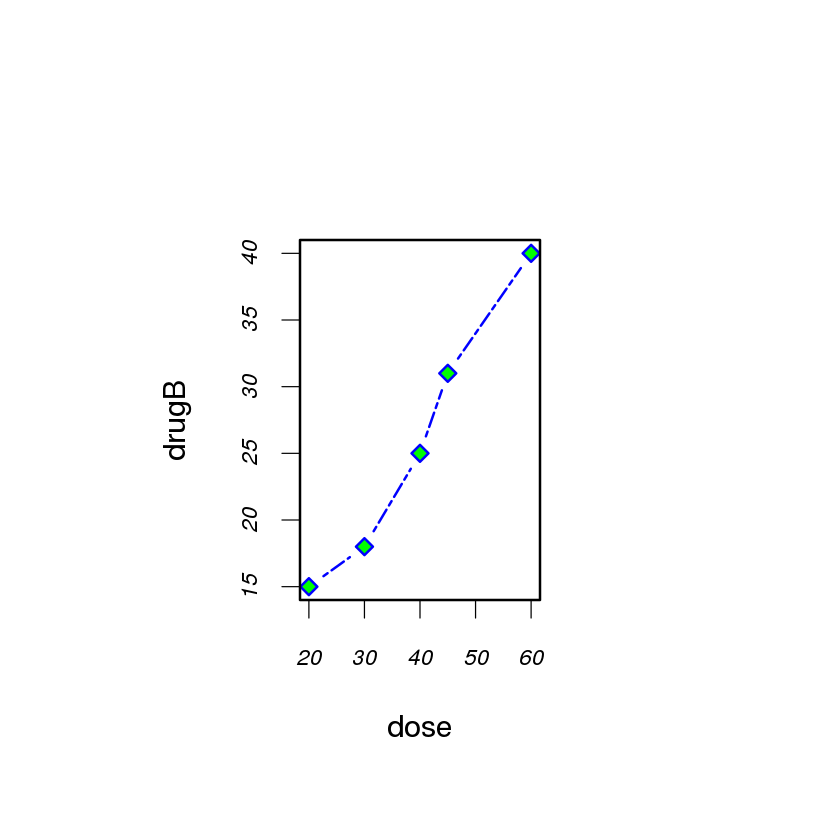Adding text, customized axes, and lengths¶

In :
#example
plot(dose, drugA, type = "b", #add data to the plot and specify the type of plot (line plot)
col = "red", lty = 2, pch = 2, lwd = 2, # col = color, lty = line type, pch = symbol, lwd = line width
main = "Clinical Trials for Drug A", #main = main title of the graph
sub = "This is hypothetical data", #sub = subtitle
xlab = "Dosage", ylab = "Drug Response", #xlab, ylab = axis labels
xlim = c(0, 60), ylim = c(0, 70)) #xlim, ylim = axis ranges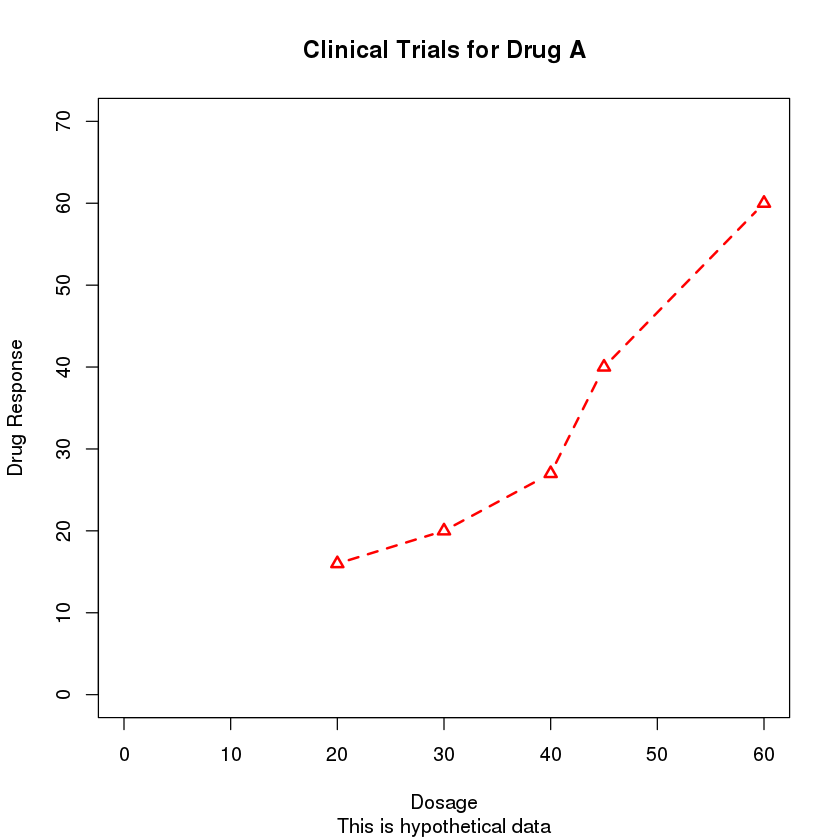In :
#install Hmisc package to enable adding of minor tick marks
install.packages("Hmisc")

Installing package into ‘/gpfs/global_fs01/sym_shared/YPProdSpark/user/s17c-9f3318fc11f06c-d37a4b9405b6/R/libs’
(as ‘lib’ is unspecified)
also installing the dependencies ‘evaluate’, ‘highr’, ‘markdown’, ‘backports’, ‘knitr’, ‘checkmate’, ‘survival’, ‘Formula’, ‘acepack’, ‘htmlTable’, ‘viridis’


In :
#invoke the Hmisc library
library(Hmisc)

In :
#another example of custom axes

#specify the data to be plotted
x <- c(1:10)
y <- x
z <- 10/x

#identify settings that can be customized
opar <- par(no.readonly = T) #save the list of settings that can be modified to the "opar" object

#increase the margins of the graph
par(mar = c(5, 4, 4, 8) + 0.1) #mar is a numerical vector indicating margin size,
#where c(bottom, left, top, right) is expressed in lines.
#the default is c(5, 4, 4, 2) + 0.1

#create the plot
plot(x, y, type = "b", #data, type = line graph
pch = 21, col = "red", #pch = symbol, col = color
yaxt = "n", lty = 3, ann = F) #yaxt = "n" suppresses the y-axis; lty = type of line; ann = F removes default titles and labels

#add a line to this plot, plotting x and z
#you use a lines() function here to add the line to the existing plot; if you used plot(), if would create a new plot
lines(x, z, type = "b", pch = 22, col = "blue", lty = 2) #type = line graph; pch = symbol; col = color; lty = type of line

#draw the axes
axis(2, #this integer indicates the side of the graph on which to draw the axis (1 = bottom, 2 = left, 3 = top, 4 = right)
at = x, #numeric vector indicating where tick marks should be drawn
labels = x, #character vector of labels to placed at the tick marks (if NULL, "at" values are used)
col.axis = "red", #line and tick mark color
las = 2) #specifies that the labels are parallel (= 0) or perpendicular (= 2) to the axis

axis(4, #this integer indicates the side of the graph on which to draw the axis (1 = bottom, 2 = left, 3 = top, 4 = right)
at = z, #numeric vector indicating where tick marks should be drawn
labels = round(z, digits = 2), #character vector of labels to placed at the tick marks; rounded off to 2 decimal places
col.axis = "blue", #line and tick mark color
las = 2, #specifies that the labels are parallel (= 0) or perpendicular (= 2) to the axis
cex.axis = 0.7, #cex.axis = text size of axis text, 0.7x normal size
tck = -0.01) #tck = length of each tick mark as a function of the plotting region
#a negative number is outside the graph, a positive number is inside
#0 suppresses ticks, and 1 creates gridlines.  The default value is -0.01

mtext("y = 10/x", #mtext() function is used to add text to the margins of the plot
side = 4, #this integer indicates the side of the graph on which to draw the axis (1 = bottom, 2 = left, 3 = top, 4 = right)
line = 3, #on which margin line; starting at 0 and counting outwards
cex.lab = 1, #cex.lab = text size of axis label, 1x normal size
las = 2, #specifies that the labels are parallel (= 0) or perpendicular (= 2) to the axis
col = "blue") #col = color

title("An Example of Creative Axes", #title of the plot
xlab = "X values", #xlab = x-axis label
ylab = "Y = X") #ylab = y-axis label

minor.tick(nx = 2, ny = 3, tick.ratio = 0.5) #nx, ny = number of intervals into which to divide the area between the major tick marks
#on the x-axis and y-axis respectively.  tick.ratio is the size of the minor tick mark
#relative to the major tick mark

#restore orginal settings
par(opar) #restore original settings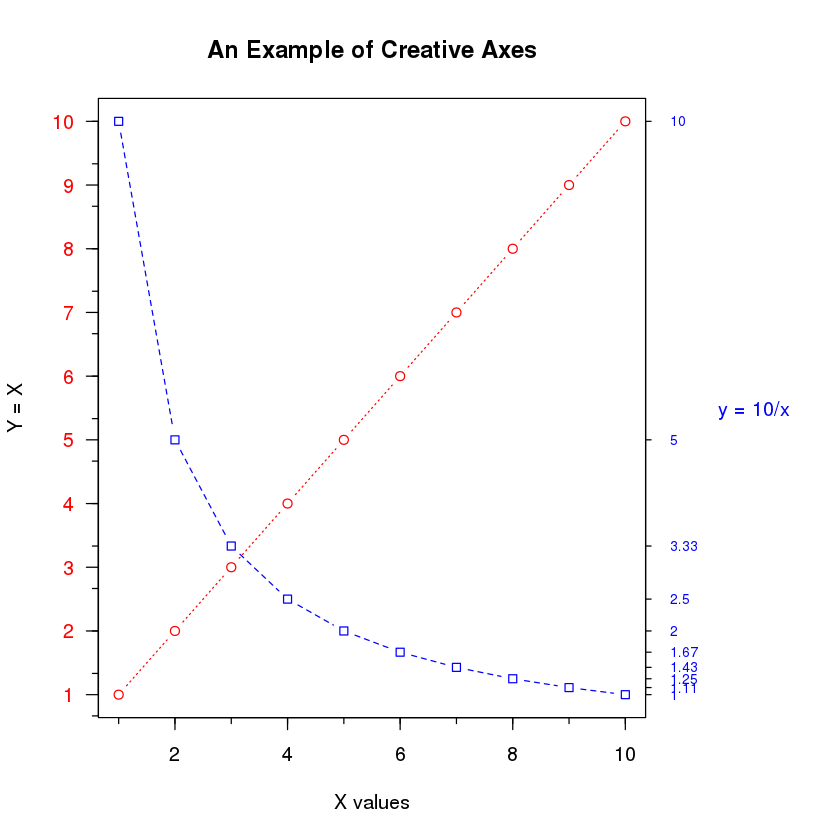In :
#example
#data
dose <- c(20, 30, 40, 45, 60) #vector with dosage levels
drugA <- c(16, 20, 27, 40, 60) #vector with drugA dosage
drugB <- c(15, 18, 25, 31, 40) #vector with drugB dosage

#identify settings that can be customized
opar <- par(no.readonly = T) #save the list of settings that can be modified to the "opar" object

#customize settings
par(lwd = 2, cex = 1.5, font.lab = 2) #lwd = line width 2x normal size; cex = plotted text, 1.5x normal size
#font.lab = font of the label, 2x normal size

#generating the graph
plot(dose, drugA, type = "b",#data, type = line graph
pch = 15, lty = 1, col = "red", ylim = c(0, 60), #pch = symbol, lty = line type (1 = continuous), col = color, ylim = y-axis limits
main = "Drug A vs. Drug B", #main title of the graph
xlab = "Drug Dosage", ylab = "Drug Response") #xlab, ylab = axis labels

#adding another line to the same graph
lines(dose, drugB, type = "b", #data, type = line graph
pch = 17, lty = 2, col = "blue") #pch = symbol, lty = line type (2 = dashed), col = color

#adding a reference line to the graph
abline(h = c(30), lwd = 1.5, lty = 2, col = "gray") #adds a reference line at y-axis = 30; lwd = 1.5x normal
#lty = line type (2 = dashed), col = color

minor.tick(nx = 3, ny = 3, tick.ratio = 0.5) #nx, ny = number of intervals into which to divide the area between the major tick marks
#on the x-axis and y-axis respectively.  tick.ratio is the size of the minor tick mark
#relative to the major tick mark

legend("topleft", inset = 0.05, title = "Drug Type", c("A", "B"), #topleft specifies where to place the legend
#inset = amount to move the legend into the graph as a fraction of the plot region
#title = character string for the legend title
#c("A", "B") = character vector with the labels for the legend
lty = c(1, 2), pch = c(15, 17), col = c("red", "blue")) #lty = vector of line styles 1 and 2; pch = vector of symbols
#col = vector of colors

#restore orginal settings
par(opar) #restore original settings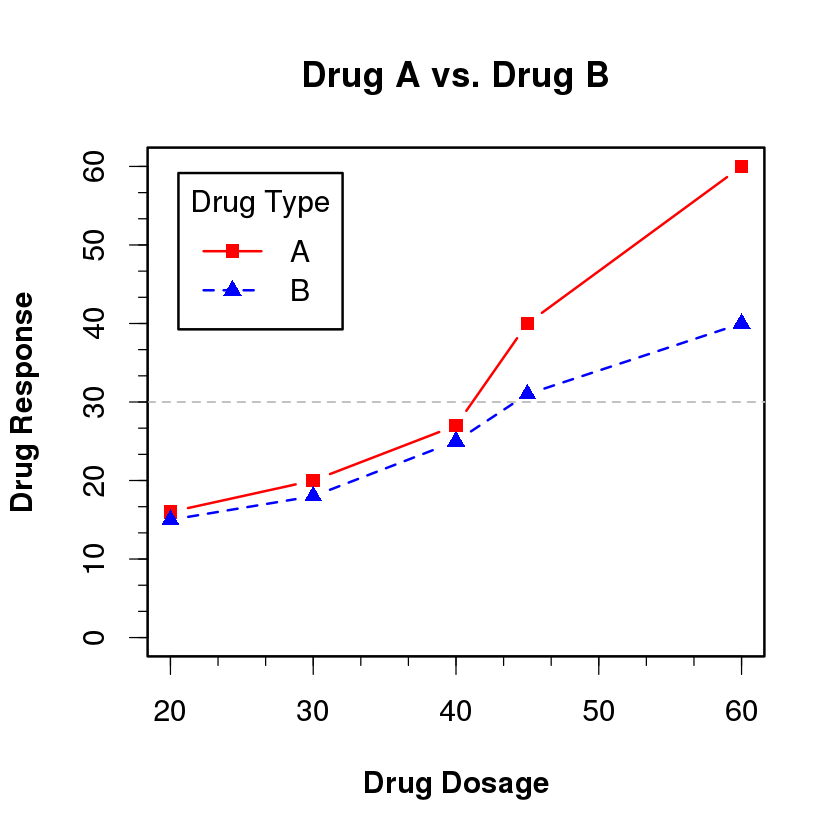Text annotations¶

In :
#example using the text() function
#text() function is used to place text within the graph
#mtext() function is used to place text within one of the four margins
#this example plots the car mileage versus care weight for the 32 automobile makes provided in the "mtcars" data frame

#attach data
attach(mtcars)

#create the plot
plot(wt, mpg, #data, wt on the x-axis and mpg on the y-axis
main = "Mileage vs. Car Weight", #main = title of the plot
xlab = "Weight", ylab = "Mileage", #x and y axis labels
pch = 18, col = "blue") #pch = symbol; col = color

text(wt, mpg, #text is placed at each (x,y) location
row.names(mtcars), #the text field is populated by the names of the cars
cex = 0.6, pos = 4, col = "red") #cex = plotted text, 0.6x normal size;
#pos = position relative to location. 1 = below, 2 = left, 3 = above, 4 = right
#col = color

mtext("This is marginal text", side = 4) #side = 4 means right margin

#detach data
detach(mtcars)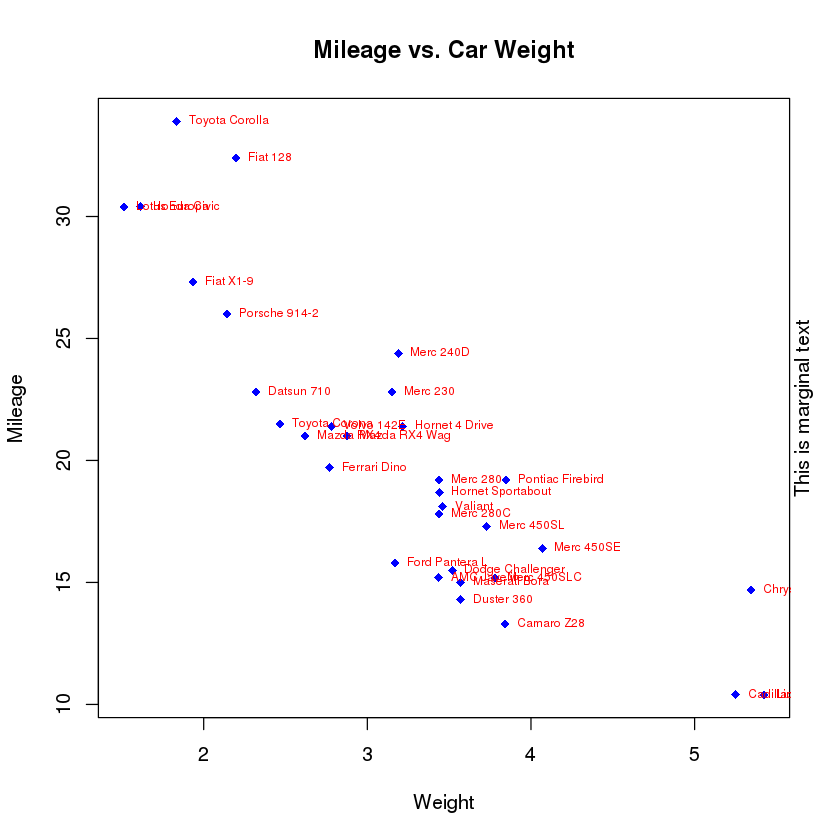In :
#another example using the text() function
#this example displays various font families

#identify settings that can be customized
opar <- par(no.readonly = T) #save the list of settings that can be modified to the "opar" object

#customize settings
par(cex = 1.5) #cex = plotted text, 1.5x normal size

#create the plot
plot(1:7, 1:7, type = "n") #x-values and y-values are the vectors 1 through 7;
#type="n" option in the plot() command is used to create the graph with
#axes, titles, etc., but without plotting the points

text(3, 3, "Example of default text") #this text is displayed at position 3,3
text(4, 4, family = "mono", "Example of mono-spaced text") #this text is displayed at position 4,4 using mono font family
text(5, 5, family = "serif", "Example of serif text") #this text is displayed at position 5,5 using serif font family

#restore orginal settings
par(opar) #restore original settings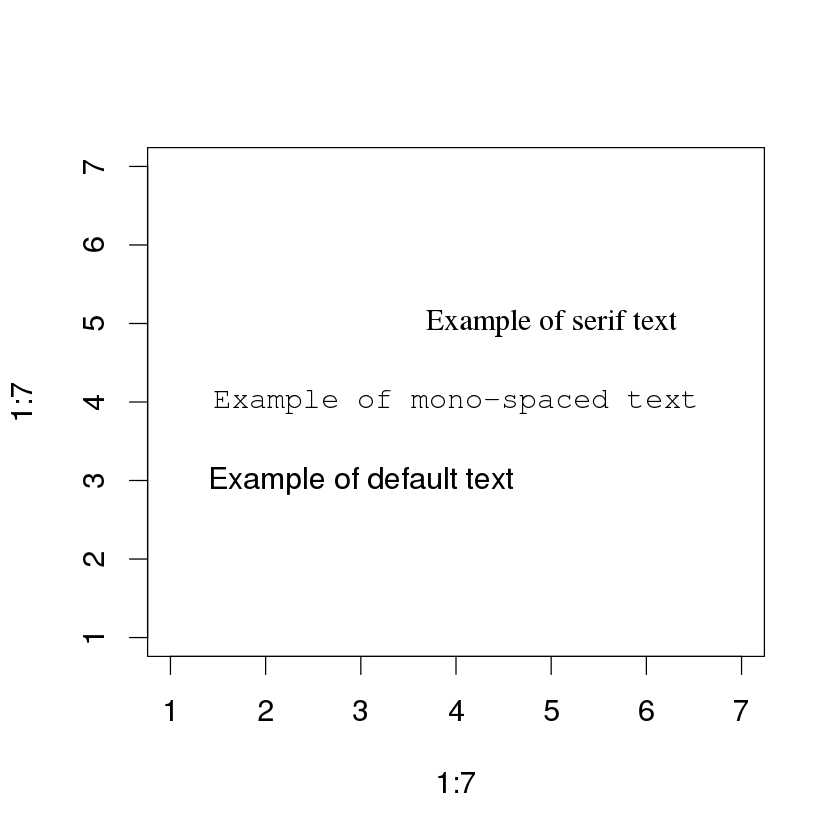Combining graphs¶

In [ ]:
#R makes it easy to combine several graphs into one overall graph using either the layout() or the par() functions
#with the par() function, you can include graphical parameter mfrow = c(nrows, ncols) to create a matrix of nrows x ncols
#plots that are filled in by row.  To fill in by column, use the mfcol = c(nrows, ncols) parameter

In :
#example: the following code creates 4 plots and arranges them into 2 rows and 2 columns

#attach data (the "mtcars" dataset)
attach(mtcars)

#identify settings that can be customized
opar <- par(no.readonly = T) #save the list of settings that can be modified to the "opar" object

#customize parameters
par(mfrow= c(2, 2)) #creates a matrix of 2 rows x 2 cols filled in by row

#create plots
plot(wt, mpg, main = "Scatterplot of wt vs mpg")
plot(wt, disp, main = "Scatterplot of wt vs disp")
hist(wt, main = "Histogram of wt") #hist = histogram
boxplot(wt, main = "Boxplot of wt")

#restore orginal settings
par(opar) #restore original settings

#detach data
detach(mtcars)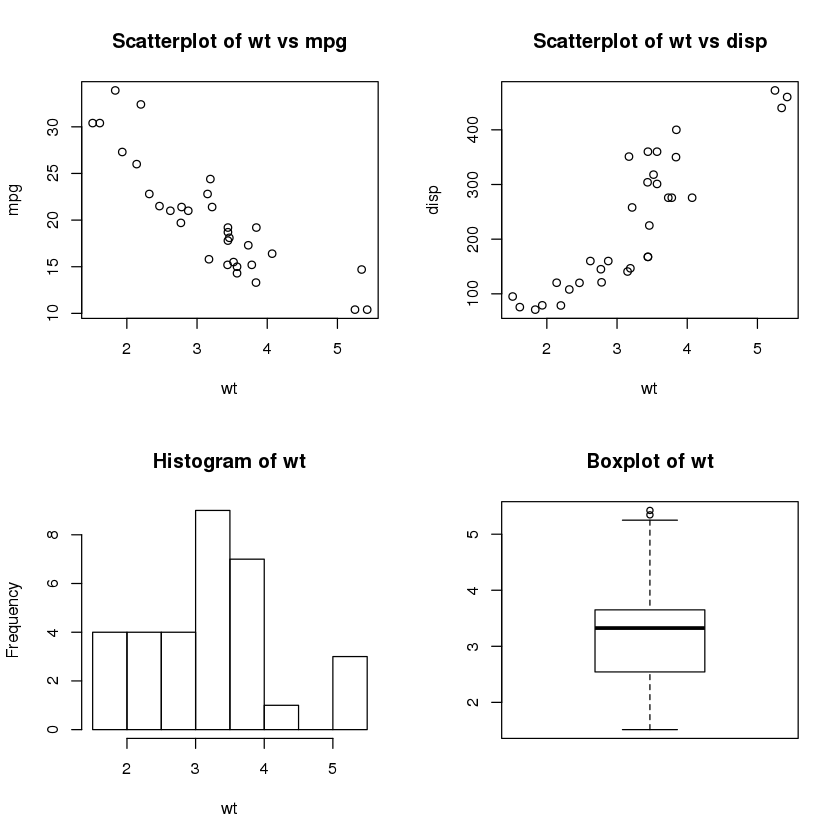In :
#another example: 3 plots in 3 rows and 1 col

#attach data (the "mtcars" dataset)
attach(mtcars)

#identify settings that can be customized
opar <- par(no.readonly = T) #save the list of settings that can be modified to the "opar" object

#customize parameters
par(mfrow= c(3, 1)) #creates a matrix of 3 rows x 1 cols filled in by row

#create plots
hist(wt)
hist(mpg)
hist(disp)

#restore orginal settings
par(opar) #restore original settings

#detach data
detach(mtcars)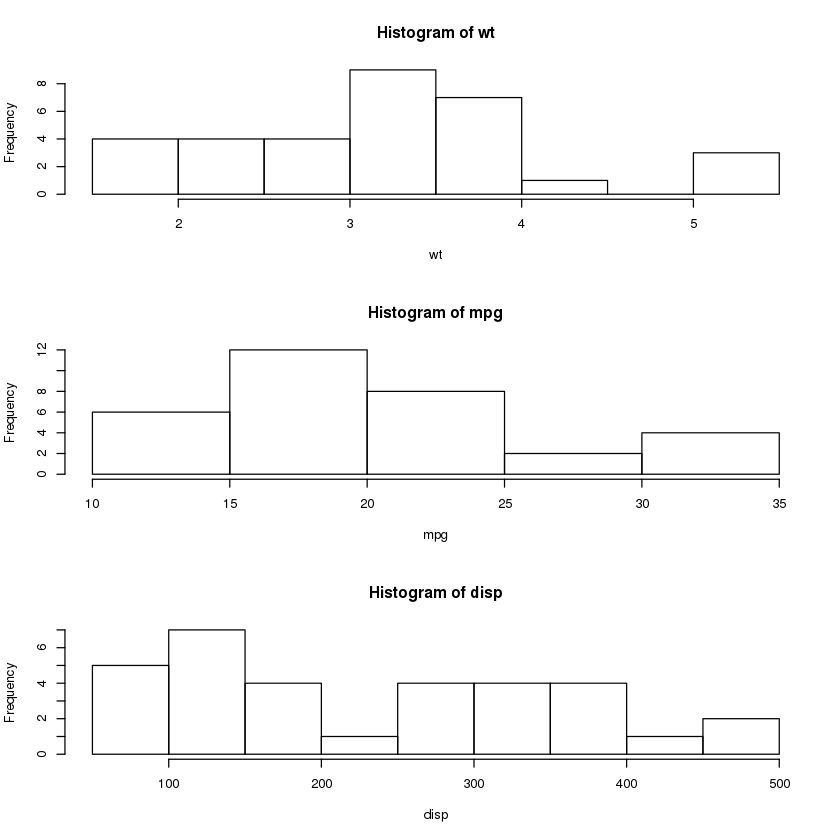In :
#example using the layout() function
#the layout() function has the form layout(mat) where "mat" is a matrix object
#specifying the location of the multiple plots to combine
#in this example, one figure is placed in row 1 and 2 figures are placed in row 2

#attach data (the "mtcars" dataset)
attach(mtcars)

#create layout
layout(matrix(c(1, 1, 2, 3), 2, 2, byrow = T)) #creates a layout that includes a matrix of 2 rows,
#with 1st row having 1 col and 2nd row have 2 cols
#byrow = T = fills in the matrix by row

#create plots
hist(wt)
hist(mpg)
hist(disp)

#detach data
detach(mtcars)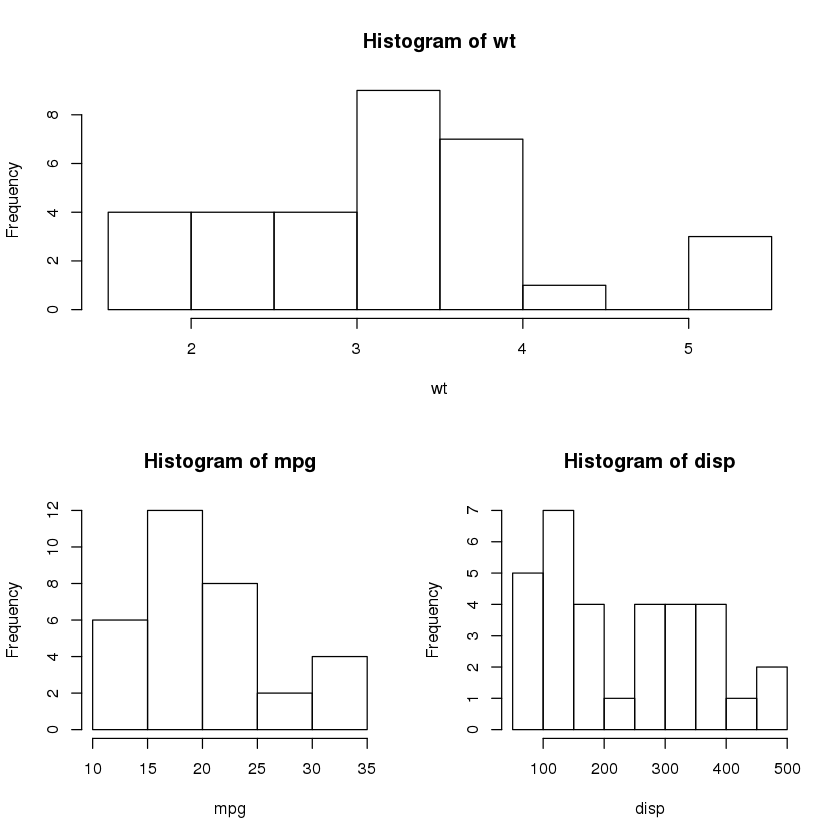In :
#example including the "widths" and "heights" options in the layout() function
#to control the size of each figure more precisely

#attach data (the "mtcars" dataset)
attach(mtcars)

#create layout
layout(matrix(c(1, 1, 2, 3), 2, 2, byrow = T), #creates a layout that includes a matrix of 2 rows,
#with 1st row having 1 col and 2nd row have 2 cols
#byrow = T = fills in the matrix by row
widths = c(3, 1), #figure in row 1 is 1/3rd the height of the figures in row 2
heights = c(1, 2)) #figure in the bottom right cell is 1/4th the width of the figure in the bottom left cell

#create plots
hist(wt)
hist(mpg)
hist(disp)

#detach data
detach(mtcars)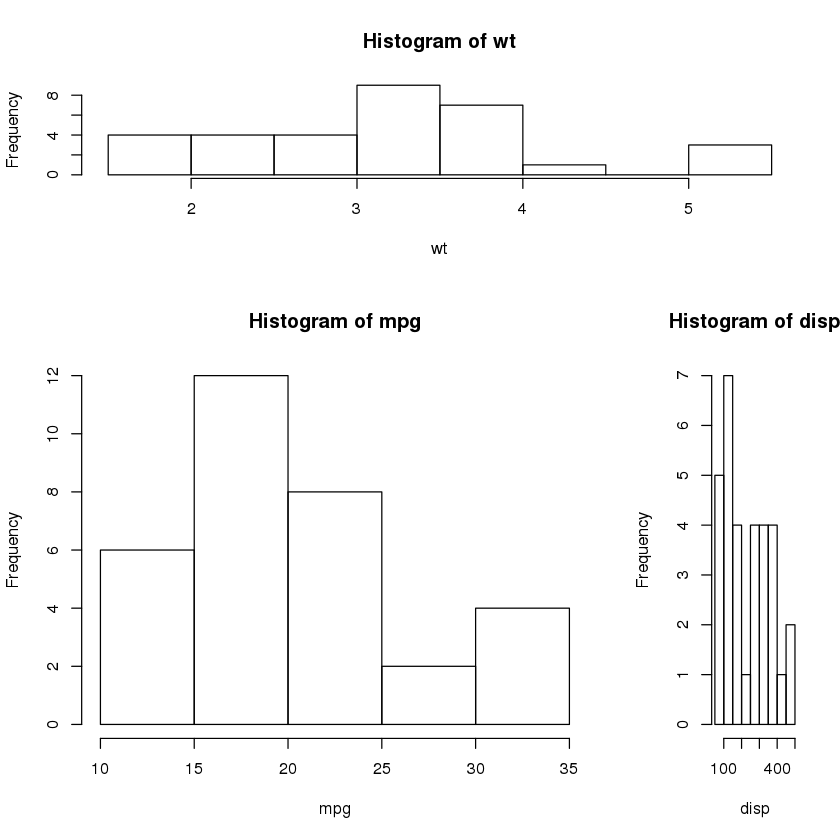Creating a figure arrangement with fine control¶

In :
#example: 2 box plots are added to a scatter plot to create a single enhanced graph

#attach data (the "mtcars" dataset)
attach(mtcars)

#identify settings that can be customized
opar <- par(no.readonly = T) #save the list of settings that can be modified to the "opar" object

#customize parameters
par(fig = c(0, 0.8, 0, 0.8)) #sets up the scatter plot going from 0 to 0.8 on the x-axis and y-axis

#create the scatter plot
plot(mpg, wt, xlab = "Miles Per Gallon", ylab = "Car Weight") #data and x, y labels

#add a box plot above the scatter plot
par(fig = c(0, 0.8, 0.55, 1), #sets up the box plot going from 0 to 0.8 on the x-axis and 0.55 to 1 on the y-axis
new = T) #adds the scatter plot to an existing plot rather than creating a new one

#create the box plot above
boxplot(mpg, horizontal = T, axes = F) #creates a boxplot of mpg horizontally (default = vertical)
#axes=FALSE suppresses both x and y axes

#add a box plot to the right of the scatter plot
par(fig = c(0.65, 1, 0, 0.8), #sets up the box plot going from 0.65 to 1 on the x-axis and 0 to 0.8 on the y-axis
new = T) #adds the scatter plot to an existing plot rather than creating a new one

#create the box plot to the right
boxplot(wt, axes = F) #creates a boxplot of wt vertically
#axes=FALSE suppresses both x and y axes